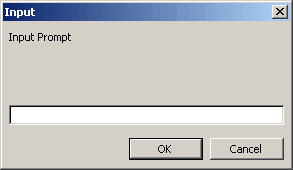# Application.InputBox

If you want to obtain information from the user you can use the Excel's InputBox Function.
This function is not to be confused with the built-in VBA.InputBox function.
There are two advantages that this function has over the VBA function:

• You can specify the datatype that is returned.

• You can detect when the Cancel key is pressed. If the Cancel button is pressed False is returned.

If you assign the return value to a Variant datatype you can easily test if this value is False.

If you just type InputBox without the Application prefix you will be using the VBA function and not the Excel one.
The value returned from the VBA function is always a string.

`sReturn = InputBox("InputBox Prompt") `

Return = InputBox(prompt [, title] [, default] [,left] [,top] [, helpfile, context] [,type])

 prompt title default left top helpfile context type

You can specify if more than one datatype is to be returned by using the sum of the corresponding type values.

### Example 1 - String

If no type is specified then a string datatype is returned.
If you press Cancel then the boolean value False is returned.

`Dim vReturn As Variant vReturn = Application.InputBox("InputBox Prompt") If vReturn = False Then End If `### Example 2 - Formula

This will accept any type of value.

`vReturn = Application.InputBox("InputBox Prompt", , , , , , , 0) `

### Example 3 - Numbers

This will only accept numbers

`vReturn = Application.InputBox("InputBox Prompt", , , , , , , 1) `

### Example 4 - Cell Reference

The user can point to cells with the mouse or type in a range directly.
The default value that is displayed is the address of the current selection.
Because this is an object you must use the Set statement to assign the range object to the returned object.
If the user clicks the Cancel button then False is returned which means the Set statement will fail generating an error.
Is Cancel is not pressed then the internal type checking of the InputBox function will guarantee a valid Range object.
The On Error Resume Next line means that any run-time error is ignored.

`Dim objRange As Range On Error Resume Next Set objRange = Application.InputBox("InputBox Prompt", , , , , , , 8) If objRange Is Nothing Then    Call Msgbox("No Range selected") Else    objRange.Select End If `

`vReturn = Application.InputBox("InputBox Prompt", , , , , , , 8) `

In VSTO this would be:

`objRange = Ctype(Application.InputBox("InputBox Prompt", , , , , , , 8),Excel.Range) `